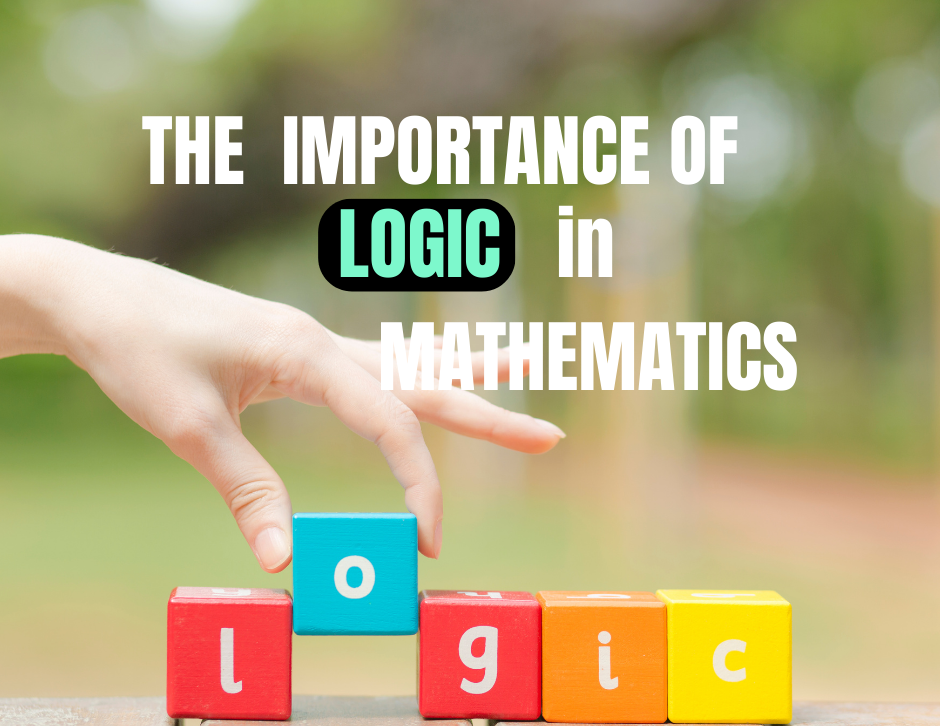top of page
Search

# The Importance of LOGIC in Mathematics

Updated: Apr 27Logic is an essential component of mathematics that forms the foundation for problem-solving and critical thinking. It is the systematic approach to reasoning that helps mathematicians arrive at accurate and reliable conclusions.

Reasoning

The importance of logic in math cannot be overstated. It is the process of using deduction to arrive at a conclusion based on a set of premises. In other words, it is the process of reasoning from known facts to new facts. Mathematics would be a collection of isolated facts and formulas that have no relation to each other without logic.

Identify Patterns and Relationships

Logic helps mathematicians to identify patterns and relationships between different mathematical concepts. With logical reasoning, mathematicians can make predictions and test hypotheses about the behavior of mathematical systems. This is particularly important in fields such as calculus, where the behavior of complex systems can be difficult to predict.

Behavior of Mathematical Systems

In addition to helping us understand the behavior of mathematical systems, logic is also crucial for the development of new mathematical concepts. By using logical reasoning, mathematicians can create new theorems and proofs that extend our understanding of mathematical concepts. This is important not only for advancing the field of mathematics, but also for its practical applications in fields such as engineering and computer science.

Accuracy of Mathematical Proofs

Logic is also essential for ensuring the accuracy of mathematical proofs. A proof is a logical argument that demonstrates the truth of a mathematical statement. Without logic, proofs would be unreliable and prone to error. By using logical reasoning, mathematicians can ensure that their proofs are rigorous and accurate.

The importance of logic in math cannot be overstated. It is the foundation for problem-solving, critical thinking, and the development of new mathematical concepts. By using logical reasoning, mathematicians can identify patterns and relationships between different mathematical concepts, make predictions and test hypotheses about the behavior of mathematical systems, and ensure the accuracy of mathematical proofs.